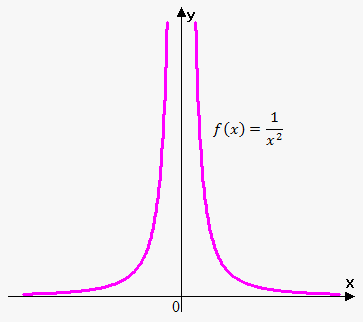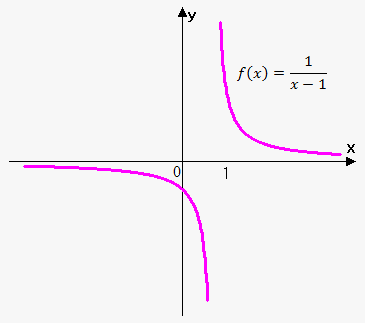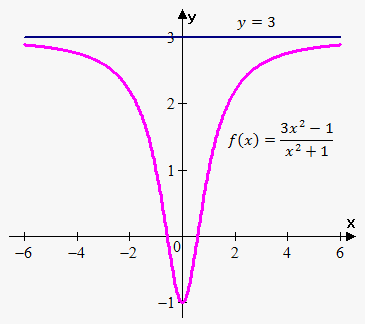# Limits Involving Infinity

## Related calculator: Limit Calculator

Now it is time to talk about limits that involve special symbol oo.

First we talk about infinite limits.

Definition. We write that lim_(x->a)f(x)=oo (lim_(x->a)f(x)=-oo) if for any E>0 there exists delta>0 such that f(x)>E\ (f(x)<-E) when |x-a|<delta.

This definition says that lim_(x->a)f(x)=oo means the following: when x approaches a f(x) increases (decreases) without a bound and can take very large number.

Once again symbol oo is just used to denote very large number. We can't perform arithmetic operations on such numbers.

Note, that we asume that lim_(x->a)f(x)=oo exists, i.e. f(x) approach very large number (infinity), denoted by oo. Unlike lim_(x->0)sin(1/x) doesn't exist, because function doesn't approach any value.

So, we use symbol oo to denote the fact that function approaches very large number, not the fact that limit doesn't exist, i.e. function doesn't approach any number.

Example 1. Find lim_(x->0) 1/x^2.When x approaches 0, x^2 also approaches 0, so, 1/x^2 approaches very large value (for example, 1/(0.001)^2=1000000), thus lim_(x->0)=1/x^2.

Also it can be easily seen that lim_(x->0)-1/x^2=-oo.

Similarly, we can define infinite one-sided limits: lim_(x->a^-)f(x)=oo, lim_(x->a^+)f(x)=oo, lim_(x->a^-)f(x)=-oo, lim_(x->a^+)f(x)=-oo.

Example 2. Find lim_(x->1)1/(x-1).When we approach 1 from the left, then x-1 is small positive number and 1/(x-1) is large negative number. Therefore, lim_(x->1^-)1/(x-1)=-oo.

When we approach 1 from the right, then x-1 is small positive number and 1/(x-1) is large positive number. Therefore, lim_(x->1^+)1/(x-1)=oo.

Since lim_(x->1^-)1/(x-1)!=lim_(x->1^+)1/(x-1) then lim_(x->1)1/(x-1) doesn't exist.

Limits at Infinity

Above we let x approach some number and the result was that values of f(x) became arbitrary large (positive or negative).

Now we let x become arbitrary large (positive or negative) and see what happens to f(x).

Example 3. Find lim_(x->oo)(3x^2-1)/(x^2+1) by guessing.If x=100 then f(100)=(3*100^2-1)/(100^2+1)~~2.9996 and if x=1000 thenf(1000)=(3*1000^2-1)/(1000^2+1)~~2.999996.

As x grows larger and larger values of f(x) become more and more close to 3.

We can make f(x) as close to 3 as we like by choosing suffciently large x.

Thus, lim_(x->oo)(3x^2-1)/(x^2+1)=3.

Similarly we can show that lim_(x->-oo)(3x^2-1)/(x^2+1)=3.

Definition. We say that lim_(x->oo)f(x)=L\ (lim_(x->-oo)f(x)=L) if for every number epsilon>0 there exists number M>0 such that |f(x)-L|<epsilon when x>M\ (x<-M).

This means that we can make f(x) as close as we like to L by taking sufficiently large x (positive or negative).

Example 4. Find lim_(x->oo)1/(x-1) and lim_(x->-oo)1/(x-1).

Observe that when x is large 1/(x-1) is small. Therefore, we can make 1/(x-1) as close to 0 as we like by taking x sufficiently large (positive or negative). Therefore, lim_(x->oo)1/(x-1)=lim_(x->-oo)1/(x-1)=0. You can see graph of function in example 2.

Example 5. Evaluate lim_(x->0^-)e^(1/x).

Let t=1/x then t->-oo as x->0^- . Therefore, lim_(x->0^-)e^(1/x)=lim_(t->-oo)e^t=0.

Again, note that oo is not a number, it is just the way to show that some value increases (or decreases) without a bound.

Example 6. Find lim_(x->oo)cos(x).

As x increases value of cos(x) oscillates infinitely many times between -1 and 1. Thus, lim_(x->oo)cos(x) doesn't exist.

Infinite Limits at Infinity

Notation lim_(x->oo)f(x)=oo means that f(x) becomes large as x becomes large. Similar meaning have following notations: lim_(x->-oo)f(x)=oo , lim_(x->oo)f(x)=-oo , lim_(x->-oo)f(x)=-oo .

For example, lim_(x->oo)e^x=oo , lim_(x->-oo)x^2=oo , lim_(x->oo)-e^x=-oo and lim_(x->-oo)x^3=-oo.

Most of the properties of the limits hold for infinite limits. Now, let's formulate a couple of laws involving infinity.

Properties of infinite limits.

Suppose a is number (can be infinity) and L is finite number.

Law 1. If lim_(x->a)f(x)=L and lim_(x->a)g(x)=oo then lim_(x->a)(f(x)+g(x))=oo.

Law 2. If lim_(x->a)f(x)=L and lim_(x->a)g(x)=oo then lim_(x->a)(f(x)-g(x))=-oo.

Law 3. If lim_(x->a)f(x)=L!=0 and lim_(x->a)g(x)=oo then lim_(x->a)(f(x)g(x))=oo if L>0 and -oo if L<0.

Law 4. If lim_(x->a)f(x)=L and lim_(x->a)g(x)=+-oo then lim_(x->a)(f(x)/g(x))=0.

Law 5. If lim_(x->a)f(x)=L and lim_(x->a)g(x)=oo then lim_(x->a)((g(x))/(f(x)))=oo if L>0 and -oo if L<0.

Law 6. If lim_(x->a)f(x)=oo and lim_(x->a)g(x)=oo then lim_(x->a)(f(x)+g(x))=oo.

Law 7. If lim_(x->a)f(x)=oo and lim_(x->a)g(x)=-oo then lim_(x->a)(f(x)-g(x))=oo.

Law 8. If lim_(x->a)f(x)=L!=0 and lim_(x->a)g(x)=0 then lim_(x->a)(f(x)/g(x))=oo if L>0 and -oo if L<0.

Law 9. If lim_(x->a)f(x)=L!=0 and lim_(x->a)g(x)=0 then lim_(x->a)(g(x)/f(x))=0.

Law 10. If lim_(x->a)f(x)=oo then lim_(x->a)cf(x)=oo where c!=0 is some constant.

Note, that these properties also hold for one-sided limits.

It is easy to believe that these properties are true.

Indeed, though we can't perform arithmetic operations over infinite values, but it is clear that sum of large numbers is large number again (Law 1), product of large numbers is again large number (Law 3), if we divide large number by very small number we will obtain again large number (Law 4) etc.

Example 7. Find lim_(x->2)(1-x)/(x-2).

Since lim_(x->2)(1-x)=-1 and lim_(x->2)(x-2)=0 then by Law 8 lim_(x->2)(1-x)/(x-2)=-oo.

Example 8. Find lim_(x->oo)(1-1/x)/(x).

Since lim_(x->oo)(1-1/x)=1 and lim_(x->oo)x=oo then by Law 4 lim_(x->oo)(1-1/x)/x=0.